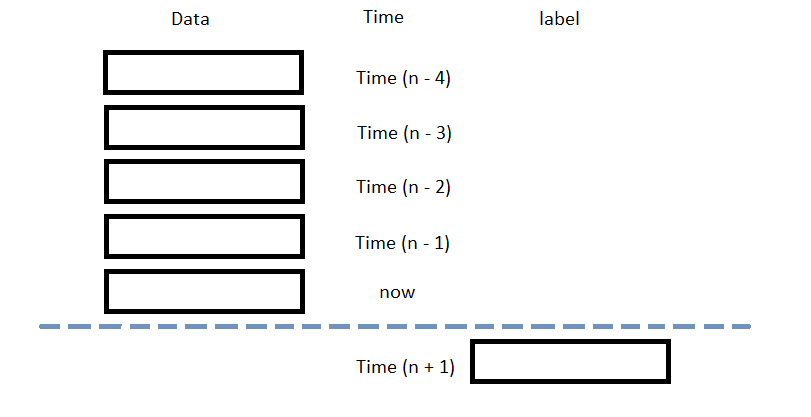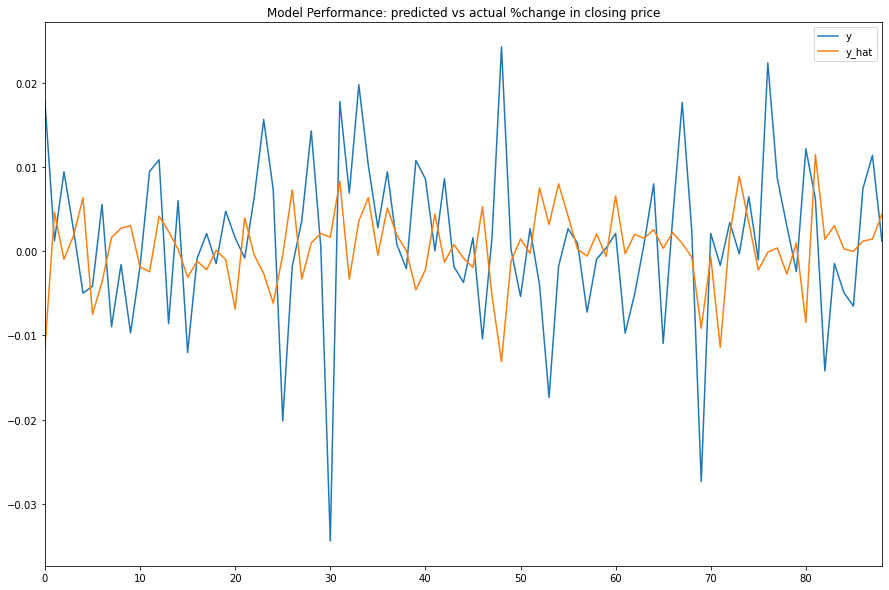# Machine Learning

## Scikit-Learn

### Introduction

This page explains how to build, train, test, and store Scikit-Learn/sklearn models.

### Import Libraries

Import the sklearn libraries.

from sklearn.svm import SVR
from sklearn.model_selection import GridSearchCV
from sklearn.model_selection import train_test_split
import joblib

You need the joblib library to store models.

### Get Historical Data

Get some historical market data to train and test the model. For example, to get data for the SPY ETF during 2020 and 2021, run:

qb = QuantBook()
history = qb.History(symbol, datetime(2020, 1, 1), datetime(2022, 1, 1)).loc[symbol]

### Prepare Data

You need some historical data to prepare the data for the model. If you have historical data, manipulate it to train and test the model. In this example, use the following features and labels:

Data CategoryDescription
FeaturesDaily percent change of the open, high, low, close, and volume of the SPY over the last 5 days
LabelsDaily percent return of the SPY over the next day

The following image shows the time difference between the features and labels:Follow these steps to prepare the data:

1. Call the pct_change method and then drop the first row.
2. daily_returns = history['close'].pct_change()[1:]
3. Loop through the daily_returns DataFrame and collect the features and labels.
4. n_steps = 5
features = []
labels = []
for i in range(len(daily_returns)-n_steps):
features.append(daily_returns.iloc[i:i+n_steps].values)
labels.append(daily_returns.iloc[i+n_steps])
5. Convert the lists of features and labels into numpy arrays.
6. X = np.array(features)
y = np.array(labels)
7. Split the data into training and testing periods.
8. X_train, X_test, y_train, y_test = train_test_split(X, y)

### Train Models

You need to prepare the historical data for training before you train the model. If you have prepared the data, build and train the model. In this example, build a Support Vector Regressor model and optimize its hyperparameters with grid search cross-validation. Follow these steps to create the model:

1. Set the choices of hyperparameters used for grid search testing.
2. param_grid = {'C': [.05, .1, .5, 1, 5, 10],
'epsilon': [0.001, 0.005, 0.01, 0.05, 0.1],
'gamma': ['auto', 'scale']}
3. Call the GridSearchCV constructor with the SVR model, the parameter grid, a scoring method, the number of cross-validation folds.
4. gsc = GridSearchCV(SVR(), param_grid, scoring='neg_mean_squared_error', cv=5)
5. Call the fit method and then select the best estimator.
6. model = gsc.fit(X_train, y_train).best_estimator_

### Test Models

You need to build and train the model before you test its performance. If you have trained the model, test it on the out-of-sample data. Follow these steps to test the model:

1. Call the predict method with the features of the testing period.
2. y_hat = model.predict(X_test)
3. Plot the actual and predicted labels of the testing period.
4. df = pd.DataFrame({'y': y_test.flatten(), 'y_hat': y_hat.flatten()})
df.plot(title='Model Performance: predicted vs actual %change in closing price', figsize=(15, 10))### Store Models

You can save and load sklearn models using the Object Store.

#### Save Models

Follow these steps to save models in the Object Store:

1. Set the key name of the model to be stored in the Object Store.
2. model_key = "model"
3. Call the GetFilePath method with the key.
4. file_name = qb.ObjectStore.GetFilePath(model_key)

This method returns the file path where the model will be stored.

5. Call the dump method with the model and file path.
6. joblib.dump(model, file_name)

If you dump the model using the joblib module before you save the model, you don't need to retrain the model.

You must save a model into the Object Store before you can load it from the Object Store. If you saved a model, follow these steps to load it:

1. Call the ContainsKey method with the model key.
2. qb.ObjectStore.ContainsKey(model_key)

This method returns a boolean that represents if the model_key is in the Object Store. If the Object Store does not contain the model_key, save the model using the model_key before you proceed.

3. Call GetFilePath with the key.
4. file_name = qb.ObjectStore.GetFilePath(model_key)

This method returns the path where the model is stored.

5. Call load with the file path.
6. loaded_model = joblib.load(file_name)

This method returns the saved model.

You can also see our Videos. You can also get in touch with us via Discord.